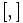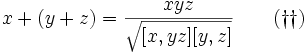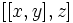# Proof of Baer construction of Lie ring for Baer Lie group

This article states and (possibly) proves a fact that is true for odd-order p-groups: groups of prime power order where the underlying prime is odd. The statement is false, in general, for groups whose order is a power of two.
View other such facts for p-groups|View other such facts for finite groups

## Statement

This statement is part of the Baer correspondence, which in turn is a special case of the Lazard correspondence.

Suppose$G$ is a Baer Lie group (?), i.e., a uniquely 2-divisible class two group. Let$[ , ]$ denote the commutator of two elements. Note that we can adopt either the left or the right convention -- the two definitions are equal because the group has class two. Denote by$\sqrt{}$ the function that takes an element and returns the unique element whose square is that element. In particular, if an element$g$ has finite order$m$, then$\sqrt{g} = g^{(m+1)/2}$ ($m$ must be odd).$G$ gets the structure of a Lie ring as follows:

Lie ring operation that we need to define Definition in terms of the group operations Further comments
Addition, i.e., define$x + y$ for$x,y \in G$$x + y := \frac{xy}{\sqrt{[x,y]}} = \sqrt{x}y\sqrt{x} = \sqrt{xy^2x}$ Since$G$ has class two,$[x,y]$ is central. Since center is local powering-invariant, applied to the prime 2, we get that$\sqrt{[x,y]}$ is central. Thus, it makes sense to divide by this element without specifying whether the division occurs on the left or on the right.
The other two definitions are equivalent, but this requires some algebraic manipulation to show. These definitions are significant because they show that the additive group operation is the same as the twisted multiplication defined for generic 2-powered groups.
Identity element for addition, denoted$0$. Same as identity element for group multiplication, denoted$e$ or$1$. This automatically follows from the way addition is defined.
Additive inverse, i.e., define$-x$ for$x \in G$. Same as$x^{-1}$, i.e., the multiplicative inverse in the group. This automatically follows from the way addition is defined.
Lie bracket, i.e., the$[ , ]$ map in the Lie ring. Same as the group commutator$[x,y] = xyx^{-1}y^{-1}$.

Further, this Lie ring is a Baer Lie ring (?): it is uniquely 2-divisible and has class at most two.

## Facts used

1. Class two implies commutator map is endomorphism

## Proof

Please see the comment, along with the definition of addition, about division by the square root being well defined.

Key proof ingredient: Fact (1).

To prove:$\! (x + y) + z = x + (y + z)$

Proof: The left side becomes:$\! (x + y) + z = \frac{\frac{xy}{\sqrt{[x,y]}} \cdot z}{\sqrt{\left[\frac{xy}{\sqrt{[x,y]}},z\right]}}$

We know that for$c$ central,$[a,bc] = [ac,b] = [a,b]$. Since the reciprocal of$\sqrt{[x,y]}$ is central, it can be dropped from inside commutator expressions, and we simplify to:$(x + y) + z = \frac{xyz}{\sqrt{[x,y]}\sqrt{[xy,z]}}$

The$\sqrt{}$ operation is a homomorphism on the center, and we can thus rewrite this as:$\! (x + y) + z = \frac{xyz}{\sqrt{[x,y][xy,z]}} \qquad (\dagger)$

Similarly, the right side of theassociativity expression we need to prove becomes:$\! x + (y + z) = \frac{xyz}{\sqrt{[x,yz][y,z]}} \qquad (\dagger\dagger)$

Thus, to prove associativity, it suffices to show that the right sides of$(\dagger)$ and$(\dagger\dagger)$ are equal, which in turn reduces to proving that:$\! [x,y][xy,z] = [x,yz][y,z]$

Now, by fact (1), the left side is$[x,y]([x,z][y,z])$, and the right side is also$([x,y][x,z])[y,z]$, and both are equal by associativity in the group, completing the proof.

Key proof ingredient: The fact that$[x,y]^{-1} = [y,x]$.

To prove:$\! x + y = y + x$

Proof: We have:$\! x + y = \frac{xy}{\sqrt{[x,y]}}$

and$\! y + x = \frac{yx}{\sqrt{[y,x]}}$

It thus suffices to prove that:$\frac{xy}{\sqrt{[x,y]}} = \frac{yx}{\sqrt{[y,x]}}$

which is equivalent to proving that:$\frac{xy}{yx} = \frac{\sqrt{[x,y]}}{\sqrt{[y,x]}}$

The left side is$[x,y]$. The right side is also$[x,y]$, which follows from the meaning of squareroot and the fact that$[y,x] = [x,y]^{-1}$.

### Agreement of identity and inverses

Key proof ingredient: The commutator vanishes when one of the elements is the identity or when the two elements are inverses of each other.

To prove: The identity element of the group is an identity element for$+$, and inverses in the group are inverses for the$+$ operation.

Proof: For this, note that if$x,y$ commute in the group, then$x + y = xy$. Now:

• Since the identity element$e$ of the group commutes with every element of the group, we have, for all$x$ in the group that$x + e = xe = x$, and similarly$e + x = ex = x$. Thus,$e$ is an identity for addition.
• Since every element commutes with its multiplicative inverse in the group, we have, for any$x$ in the group, that$x + x^{-1} = xx^{-1} = e$, which is the identity element for the group and hence also for$+$. Similarly,$x^{-1} + x$ is the identity element for the group, and hence also for$+$.

### The Lie bracket is additive in the first variable

To prove:$[x+y,z] = [x,z] + [y,z]$

Proof: We have:$\! [x+y,z] = [\frac{xy}{\sqrt{[x,y]}},z] = [xy,z] = [x,z][y,z]$

Also:$\! [x,z] + [y,z] = \frac{[x,z][y,z]}{\sqrt{[[x,z],[y,z]]}} = [x,z][y,z]$

Thus, we obtain$[x+y,z] = [x,z] + [y,z]$.

### The Lie bracket is additive in the second variable

This is analogous to additivity in the first variable, as shown above.

### The Lie bracket is alternating

This follows from the fact that the commutator of any element with itself is the identity element.

### The Lie bracket satisfies the Jacobi condition and gives a class two Lie ring

Since the Lie bracket coincides with the commutator, and the commutator satisfies that$[[x,y],z]$ is trivial for all$x,y,z$, the Lie bracket also satisfies the same condition. Thus, it satisfies Jacobi's identity and also the condition for class two.